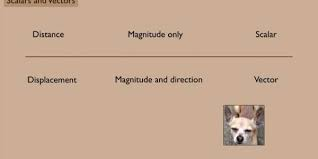The Learning Edge

(Introduction to Physics) Scalars and Vectors

(Introduction to Physics) Scalars and VectorsDistance is a scalar, while displacement is a vector.
Video Rating: / 5

Share: